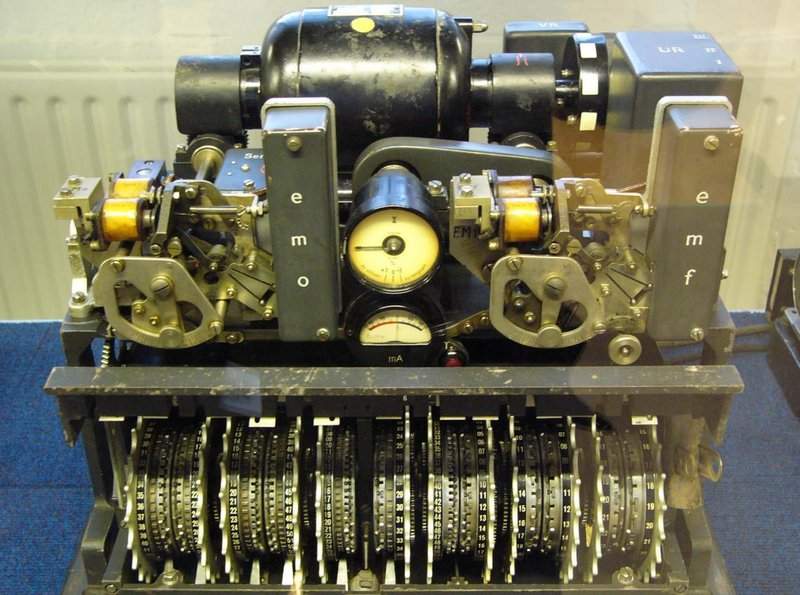Number Theory and CryptographyThese lessons are intended for mathematically interested high school students to give them an introduction to Number Theory and Cryptography. Each lesson includes two problem sets that are essential to work through. Solutions and hints to these problems can be found in section VII. In the case that these lessons are being used in a classroom setting, some of them will require multiple session to cover, the problem sets then could be used as group work or homework sets. These lessons should be covered in order. For more information and references from which the material came, links and books are suggested at the end of each lesson.

This work was made possible due to a grant from the National Science Foundation.